Function Repository Resource:

# Intrinsic3DCurve

Plot intrinsic curves in 3D

Contributed by: Enrique Zeleny
 ResourceFunction["Intrinsic3DCurve"][κ,τ,a,b,w,ds,n] plot an intrinsic curve with curvature κ and torsion τ with parameters a and b and width w in discrete steps ds applied n times.

## Details and Options

The French mathematicians Joseph Alfred Serret and Jean Frédéric Frenet found a way to represent a parametrized curve by intrinsic equations.
At each point of the curve (parametrized by arc length), three mutually perpendicular unit vectors are defined (called a TNB frame). The tangent shows the direction of motion of the point, the normal points toward the direction in which the curve bends and the binormal is a vector perpendicular to both.
Another two quantities are introduced: curvature to measure how quickly the curve is changing its direction and torsion to measure how quickly the curve is leaving the TN plane.
The starting point is {0,0,0}.
Options are the same as Graphics3D.

## Examples

### Basic Examples (4)

A simple curve:

 In:=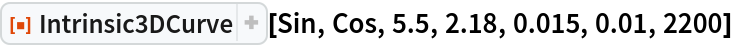Out=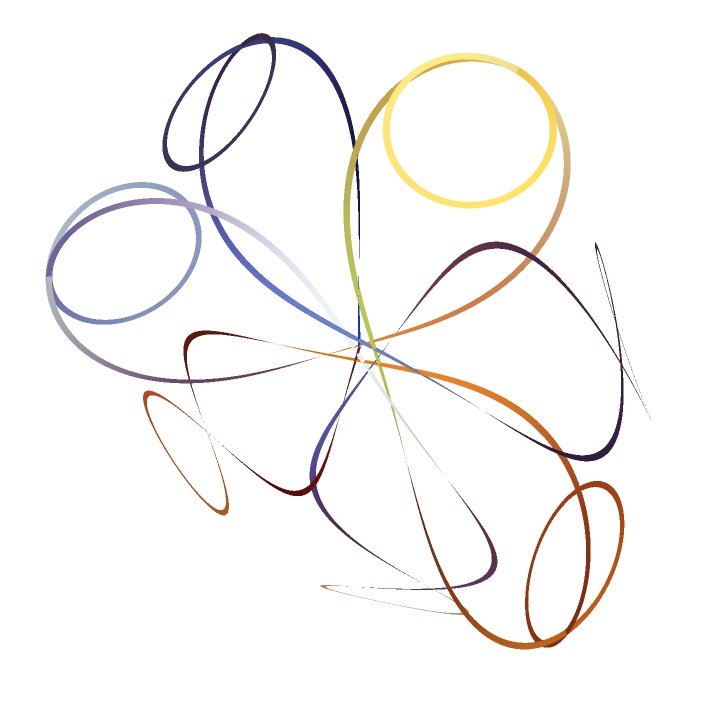Increasing width, we get a ribbon-like surface (in fact, a ruled surface—the other edge has a displaced TNB frame):

 In:=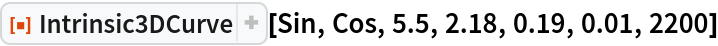Out=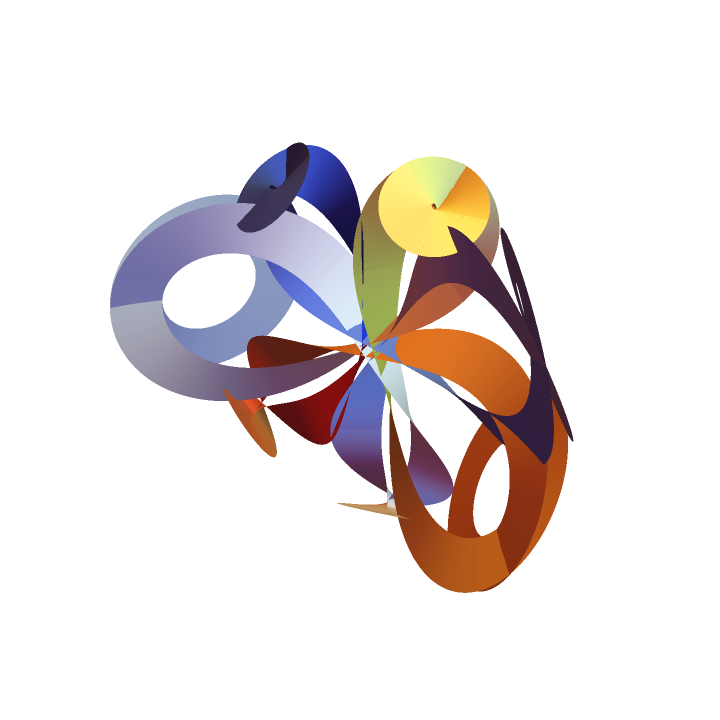With a constant function:

 In:=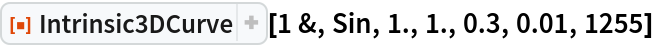Out=A higher constant function:

 In:=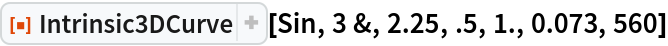Out=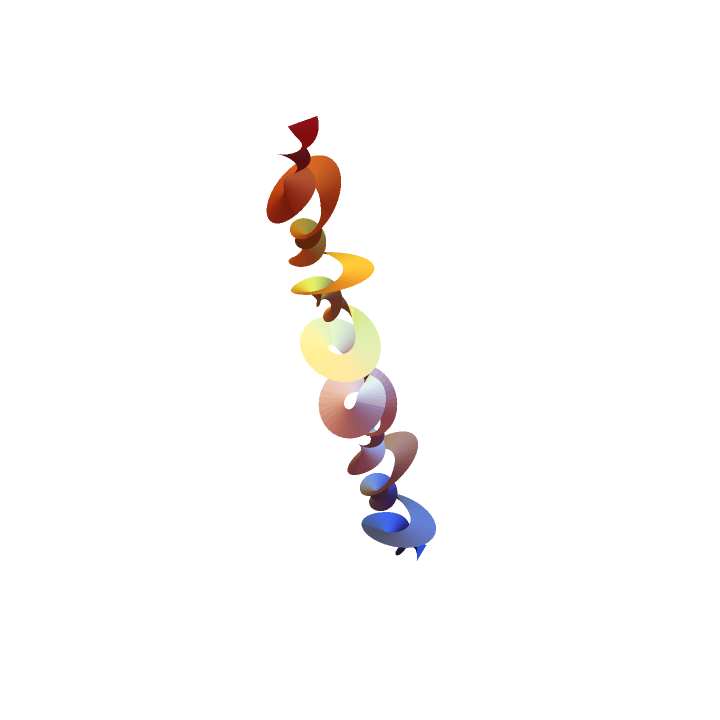### Possible Issues (2)

A function varying rapidly needs small discrete steps to plot smoothly:

 In:=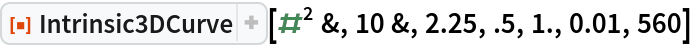Out=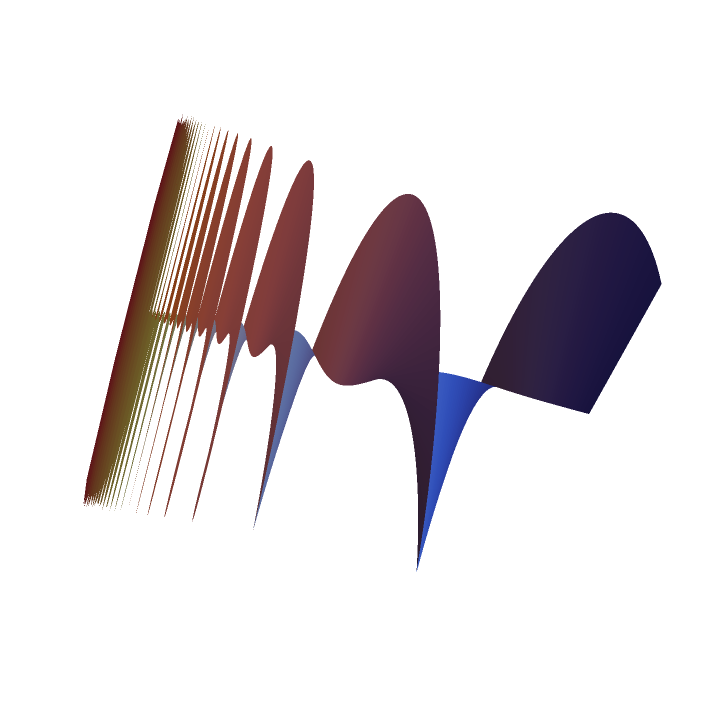Avoid undefined values like log(0):

 In:=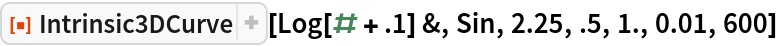Out=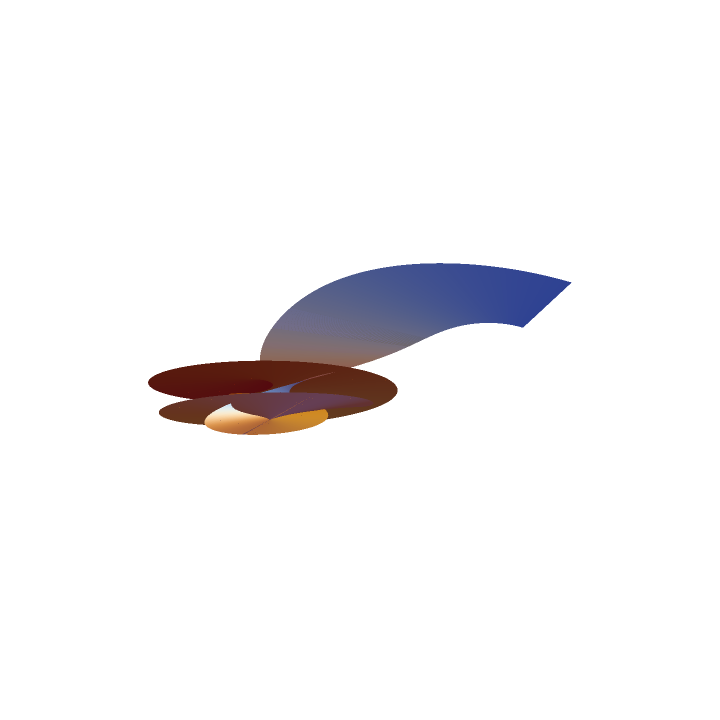## Requirements

Wolfram Language 11.3 (March 2018) or above

## Version History

• 1.0.0 – 07 March 2019